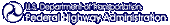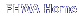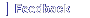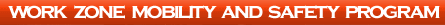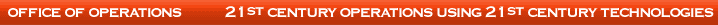# Text from 'Economic Analysis: Applications to Work Zones' PowerPoint Presentation

## Slide 1

Economic Analysis: Applications to Work Zones

Graphic: U.S. Department of Transporation logo

## Slide 2

Economic Analysis

What Is It?

• Benefits and/or costs of competing investment options are compared in common unit of the dollar
• Makes non-like performance measures comparable

## Slide 3

Economic Analysis

• Why? Performance
• What? Greatest net benefit
• When? Optimal timing
• Where? Best alignment
• How? Best implementation strategy

## Slide 4

Economic Analysis

Issues and Concepts

• Costs and benefits can be valued in dollars
• Project life cycle is basis for comparison
• To be compared, dollars in different years must be "discounted" to their present value amounts

## Slide 5

Economic Analysis

Bar Graph: Typical Life Cycle Profile

Speaker Notes:

The above graph is a fairly typical profile of the monetized cost and benefit flows of a transportation project. The initial cost spike represents the capital cost of the project. Subsequent costs are attributable to maintenance (smaller bars) and periodic rehabilitations (medium height bars). Benefits build over time as traffic grows, due in large part to the effect of the project in reducing future congestion and delay.

## Slide 6

Economic Analysis

The present value represents one divided by the sum of 1 plus r raised to the power of t multiplied by A at time t

where
PV = present value at time zero (base year)
r = discount rate
t = time (number of year)
A = amount of benefit or cost in year t

Speaker Notes:

The formula above is the most basic calculation of present value. The term which incorporates the discount rate "r" is called the discount factor. Multiplying a future sum by the appropriate discount factor for that future year will yield the present value of that sum at time zero.

## Slide 7

Economic Analysis

Example of Discounting

• What if we want to determine how much a \$1,000 benefit in 30 years is worth to us today?
• \$1000 is in "real" dollars (i.e., in dollars with today's purchasing power)
• Discount rate is 3%

Speaker Notes:

Application of the discounting formula is easy to demonstrate. In this example, we want to learn what a \$1,000 in resources to be realized 30 years from now would be worth to us today at a 3% discount rate. The substitution of these values into the discounting formula is shown on the next slide.

## Slide 8

Economic Analysis Example (continued)

Plug values into discounting formula:

The present value represents one divided by the sum of 1 plus .03 raised to the 30 power multiplied by \$1,000 (at 30 years)

Do calculations:

PV equals \$1,000 multiplied by 0.41199

This equals \$412.

Speaker Notes:

The bracketed term in the above equation is often referred to as the discount factor. In our example is equal to 1/(1.03)^30 or 0.41199. When \$1,000 is multiplied by this amount, the present value of \$1,000 in 30 years is revealed to be \$412 in today's dollars.

## Slide 9

Economic Analysis

Discount Rate Is Important

• Higher the discount rate, the lower the present value of a future dollar
• At 3%, \$1,000 30 years from now is worth only \$412 today
• Worth \$231 at 5% and \$57 at 10%
• Discount rate can influence project selection or design

## Slide 10

EA Methods

• Benefit-Cost Analysis
• Life-Cycle Cost Analysis

## Slide 11

EA Methods

Benefit Cost Analysis (BCA)

• BCA compares discounted value of project's benefits to discounted value of its costs
• The blue and red bars on the life cycle profile
• BCA is different from financial analysis, which focuses on how to fund a project

## Slide 12

EA Methods

BCA Formula

• BCA is done using the basic multi-year discounting formula where

PV = present value

t=time

N=number of interest periods

r=interest rate per period

The present value represents the sum of all values of the reciprocal of 1 plus r raised to the power of t, multiplied by the difference between the benefit and cost at time t, for all times t between zero and N inclusive.

Speaker Notes:

The above formula shows the BCA formula, which is a direct expansion of the multi-year discounting formula discussed in the module on economic fundamentals. The formula is applied to each design alternative or project being evaluated for identical analysis periods.

## Slide 13

EA Methods

Applications of BCA

• Project-level analysis
• Selecting ITS or operations technologies
• Highway program-level analysis
• Regulatory analysis

## Slide 14

EA Methods

Life-Cycle Cost Analysis (LCCA)

• Subset of BCA
• The "blue bars" on the life cycle profile
• LCCA reveals lowest life-cycle cost alternative for a project
• Used only when all design alternatives yield same benefits

## Slide 15

EA Methods

LCCA Formula where "Cost" equals the cost for design alternative in year t

The present value represents the sum of all values of the reciprocal of 1 plus r raised to the power of t, multiplied by the cost at time t, for all times t between zero and N inclusive.

## Slide 16

EA Methods

Applications of LCCA

• Evaluation of pavement preservation strategies
• Project planning and implementation, especially the use and timing of work zones
• Value Engineering

## Slide 17

EA Methods

How to Get Best LCCA Results

• Evaluate all reasonable design alternatives for the project
• Analyze alternatives over identical analysis periods
• Evaluate all relevant costs that vary among the alternatives

## Slide 18

EA Methods

Cost Items Used in LCCA

Agency Costs

• Design and engineering
• Land acquisition
• Construction
• Reconstruction/Rehabilitation
• Preservation/Routine Maintenance

User Costs At Work Zones

• Delay
• Crashes
• Vehicle Operating

## Slide 19

EA Methods

Valuation of User Time

• Business travel valued at wage plus benefits
• Personal travel valued at what travelers are willing to pay to reduce travel time
• Usually a percentage of wage
• People do value their time

## Slide 20

EA Methods

Inclusion of User Costs in EA

• Some agencies resist valuation of user delay caused by construction
• However, agencies seeking to reduce work zone impacts without user cost data may overspend or underspend

## Slide 21

• Other tools increase the usefulness of BCA and LCCA
• Traffic Forecasting
• Risk Analysis
• Economic Impact Analysis

## Slide 22

Traffic Forecasting

• Queuing models
• Included in RealCost LCCA Software
• Traffic simulation models
• Corsim
• QuickZone
• Travel demand models

## Slide 23

Risk Analysis

• Uncertainty can be measured and mitigated
• Sensitivity and probabilistic methods
• Risk can be mitigated using alternative engineering, contractual methods, etc.

## Slide 24

Linking EA to Other Tools Economic Impact Analysis

• EA focuses on direct benefits and costs of highway projects
• Time savings, safety, externalities
• EIA "translates" EA results into indirect economic effects
• Delays affect business and jobs
• Not additive to value of direct benefits and costs

## Slide 25

LCCA Applied to Work Zones

• LCCA can be used to compare construction/work zone mitigation strategies
• FHWA's RealCost LCCA software can measure agency costs (construction, rehabilitation, maintenance) and user costs over multi-year periods

## Slide 26

LCCA Applied To Work Zones

Mitigation Strategies

• There are many ways to mitigate construction impacts
• TMP and work zone strategies
• Innovative contracting
• Design features and materials
• Does value of mitigation justify costs?

## Slide 27

LCCA Applied To Work Zones

Comparing Strategies

• Each construction/WZ strategy involves trade-offs
• Agency vs. user costs
• Initial vs. long-term costs
• LCCA approach permits comparison of cost trade-offs

## Slide 28

Application to Work Zones Example

• Consider Stone Matrix Asphalt (SMA) vs. Superpave (SP), each with 24 hour vs. nighttime work zones
• 5 mile, 4 lane road mill & fill
• 25,000 vehicles Average Daily Traffic, rising to 60,000 ADT in 35 years
• One lane closed each way

## Slide 29

Application to Work Zones

Example (Continued)

• ADT is allocated by RealCost model to peak/off-peak times
• RealCost model calculates user delay caused by work zones
• 35 year analysis period
• 4 percent real discount rate

## Slide 30

Application to Work Zones Example (Continued)

• SMA costs 20 percent more than SP per overlay but lasts longer 20 percent longer between rehabilitations
• Nighttime work zones increase agency cost by 10 percent

## Slide 31

LCCA Applied To Work Zones

Example Results

Costs Over 35 Years in \$1000
WZ Strategies Agency Present Value User Present Value
SP - 24 Hr WZ \$2,605 \$4,969
SMA - 24 Hr WZ \$2,721 \$3,715
SP - Night Only \$2,865 \$1,226
SMA - Night Only \$2,987 \$1,055

## Slide 32

LCCA Applied To Work Zones

Example Results (Continued)

• Least cost option for the agency (SP/24 hours) is highest cost for travelers
• Using SMA reduces traveler cost due to fewer rehabs
• Nighttime work zones eliminate most of delay for SP and SMA at little additional cost to the agency

## Slide 33

For Further Information

Economic Analysis Primer
FHWA IF-03-032, August 2003

Graphic: Cover of Economic Analysis Primer book

Contents:

• Economic Fundamentals
• Life-Cycle Cost Analysis
• Benefit-Cost Analysis
• Forecasting Traffic
• Risk Analysis
• Economic Impact Analysis

## Slide 34

For Further Information

Life-Cycle Cost Analysis Materials

RealCost Software and workshops-call your Division Office

Graphics: Covers of Life-Cycle Cost Analysis Materials

## Slide 35

For Further Information

Other Economic Materials

FHWA's Office of Asset Management, Evaluation and Economic Investment Team:
www.fhwa.dot.gov/infrastructure/asstmgmt/invest.htm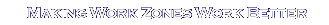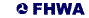US DOT Home | FHWA Home | Work Zone Home | Operations Home | Privacy Policy